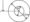# Fluid Mechanics Feb 2011

Unit-I

1. a) Differentiate between:)

i) Liquids and gases

ii) Real fluids and Ideal fluids

iii) Specific weight and specific volume.

iv) Dynamic viscosity and Kinematic viscosity.

b) Two plates are placed at a distance of 0.15 mm apart. The lower plate is fixed while the upper plate having surface area 1.0 m2 is pulled at 0.3 m/sec. Find the force and power required to maintain this speed, if the fluid separating them is having viscosity 1.5 poise.

OR

a)  Define the surface tension. Prove that the relationship between surface tension and pressure inside a droplet of liquid in excess of outside pressure is given by /?=— where ct surface tension and d is diameter of droplet.

b)  Determine the bulk modulus of elasticity of a liquid, if the pressure of liquid is increased from 70 N/cm2 to 130 N/cm2. The volume of the liquid decreases by 0.15 percent.

Unit -II

2. a) Define pressure. Obtain an expression for pressure intensity at a point in a fluid. State and prove Pascal’s law.150 cm

mercury (sp.gravity 13.6)

(b) lank The tank shown in figure is closed at top and contains air at a pressure pA. Calculate the value of pA for the manometer reading.

OR

a)  Derive an expression for the depth of centre of pressure from free surface of liquid of an inclined plane surface submerged in liquid.

b)  A circular annular area of 2.0 m outer diameter and 1.0m inner diameter is immersed vertically in water with the centre of the area at 3.0 m below the water surface find

i) the force exerted on one side of the area, and

ii) the location of the centre of pressure.Unit-III

3 (a)Distinguish between

ii)      Uniform and non-uniform flow

iii)     Compressible and Incompressible flow

iv)     Rotational and irrotational flow.

(b) What do you understand by

i)       Total acceleration

ii)      Convective acceleration

iii)     Local acceleration

iv)     Velocity potential and stream function.

OR

(a)Define the equation of continuity. Obtain an expression for continuity equation for a three-dimensional flow.

(b)If for a two-dimensional potential flow, the velocity potential is given by ^=4x(3_y-4), determine the velocity at the point (2,3). Determine also the value of stream function y/ at the point (2,3).

Unit-IV

4 (a)Explain the principle of venturi-meter with a neat sketch. Derive the expression for the rate of flow of fluid through it.

(b)Water is flowing through a pipe having diameters 20 cm and 15 cm at sections 1 and 2 respectively. The rate of flow through pipe is 40 litre/sec. The section 1 is 6 m above datum line and section 2 is 3 m above the datum. If the pressure at section 1 is 29.43 N/cm2, find the intensity of pressure at section 2.

OR

(a)Prove that the discharge through a triangular notch or weir is given by £?=y^cd tan.^y[2g H% Where H = head of water over the notch or weir.

= angle of notch or weir

b)  A circular tank of diameter 4 meter contains water upto a height of 5 m. The tank is provided with an orifice of diameter 0.5 m at the bottom. Find the time taken by water for completely emptying to take. Take cd – 0.6.

Unit-V

5. a) How will you determine the loss of head due to friction in pipes by using

i) Darcy formula and

ii) Chezy’s formula.

Define the hydraulic gradient line and total energy line.

b)  Find the diameter of a pipe of length 2000 m when the rate of flow of water through the pipe is 200 lit/sec and the head lost due to friction is 4 m. Take the value of C = 50 in Chezy’s formula.

OR

a)  Find an expression for the power transmission through pipes. What is the condition for max imum transmission of power and corresponding efficiency of transmission.

b)  Three pipes of lengths 800 m, 600 m and 300 m and of diameters 400 mm, 300 mm and 200 mm respectively are connected in series. The ends of compound pipe is connected to two tanks, whose water surface levels are maintained at a difference of 15 m. Determine the rate of flow of water through the pipes iff= 0.005.

### 1 thought on “RTU Previous Year Question Papers BE CE Third Semester Fluid Mechanics Feb 2011”

1.very nice